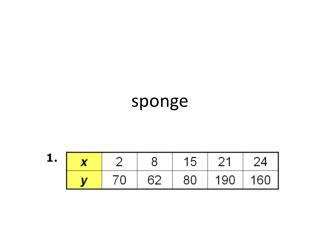DownloadDownload Presentationsponge

# sponge

Download Presentation## sponge

- - - - - - - - - - - - - - - - - - - - - - - - - - - E N D - - - - - - - - - - - - - - - - - - - - - - - - - - -
##### Presentation Transcript

1. sponge

2. Example 1B: Using Finite Differences to Determine Degree Use finite differences to determine the degree of the polynomial that best describes the data. The x-values increase by a constant 3. Find the differences of the y-values. First differences: 25 10 15 37 73 Not constant Second differences: –15 5 22 36 Not constant Third differences: 20 17 14 Not constant Fourth differences: –3 –3 Constant The fourth differences are constant. A quartic polynomial best describes the data.

3. Check It Out! Example 1 Use finite differences to determine the degree of the polynomial that best describes the data. The x-values increase by a constant 3. Find the differences of the y-values. First differences: 20 6 0 2 12 Not constant Second differences: –14 –6 2 10 Not constant Third differences: 8 8 8 Constant The third differences are constant. A cubic polynomial best describes the data.

4. Probability rules • 1. Any probability is a number between 0 and 1. • 2. The sum of the probabilities of all possible outcomes must equal 1. • 3. If two events have no outcomes in common, the probability that one or the other occurs is the sum of their individual probabilities. • 4. The probability that an event does not occur is 1 minus the probability that the event does occur.

5. Distance learning • P(40 or over) = • P(not 18 to 23) = • P(30 or over) =

6. Benford’s law • http://www.youtube.com/wat • ch?v=O8N26edbqLM